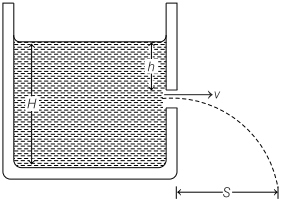# Torricelli’s Theorem Formula and Derivation – Hydrodynamics

Torricelli’s theorem, is a theorem in fluid dynamics relating the speed of fluid flowing from an orifice to the height of fluid above the opening.

We are giving a detailed and clear sheet on all Physics Notes that are very useful to understand the Basic Physics Concepts.

## Torricelli’s Theorem Formula and Derivation – Hydrodynamics

Torricelli’s Theorem
Velocity of efflux (the velocity with which the liquid flows out of a orifice or narrow hole) is equal to the velocity acquired by a freely falling body through the same vertical distance equal to the depth of orifice below the free surface of liquid.Torricelli’s Law Formula:

Velocity of efflux, v = $$\sqrt{2 g h}$$

where,
h = depth of orifice below the free surface of liquid.

t = $$\sqrt{\frac{2(H-h)}{g}}$$
Horizontal range, S = $$\sqrt{4 h(H-h)}$$

where, H = height of liquid column.

Horizontal range is maximum, equal to height of the liquid column H, when orifice is at half of the height of liquid column.
If the hole is at the bottom of the tank, then time required to make the tank empty is

t = $$\frac{A}{A_{0}} \sqrt{\frac{2 H}{g}}$$

where, A is area of the container and Ao is area of orifice.
Volume of liquid coming out from the orifice per second

= VAo = Ao$$\sqrt{2 g h}$$

Hydrodynamics:
In physics, hydrodynamics of fluid dynamics explains the mechanism of fluid such as flow of liquids and gases. It has a wide range of applications such as evaluating forces and momentum on aircraft, prediction of weather, etc.